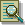The TIMEPLOT Procedure

# Example 4: Superimposing Two Plots

Procedure features:

PROC TIMEPLOT statement options:

 MAXDEC=

PLOT statement arguments:

 using two types of plot requests OVERLAY REF=MEAN(variable(s)) REVERSE
Data set: SALES

This example

• superimposes two plots on one set of axes

• specifies a variable to use as the plotting symbol for one plot and a character to use as the plotting symbol for the other plot

• draws a reference line to the mean value of each of the two variables plotted

• reverses the labeling of the axis so that the largest value is at the far left of the plot.`options nodate pageno=1 linesize=80 pagesize=60;``proc timeplot data=sales maxdec=0;`` plot stove=seller icebox='R' /`` overlay`` ref=mean(stove icebox)`` reverse;`` label icebox='Refrigerators';```` title 'Weekly Sales of Stoves and Refrigerators'; title2 'for the'; title3 'First Six Weeks of the Year'; run;`````` Weekly Sales of Stoves and Refrigerators 1 for the First Six Weeks of the Year Stove Refrigerators max min 3550.43 184.24 *--------------------------------------------------* 1313 3451 |R | K | | 728 2520 | | R | L | 222 3241 | R | | K | 184 2675 | | R | L| 2263 3160 | R | K | | 267 2805 | | R | L | 1787 3400 | R | K | | 275 2871 | | R | L | 2910 3550 |R | K | | 398 2730 | | R | L | 820 3386 | R | | K | 2242 2671 | | R L | | *--------------------------------------------------* ```Previous Page | Next Page | Top of Page